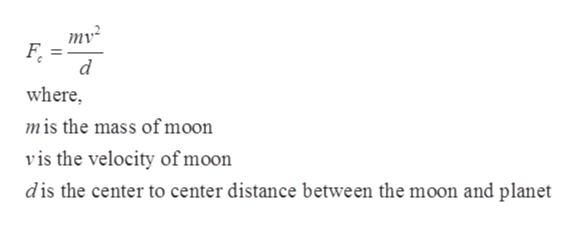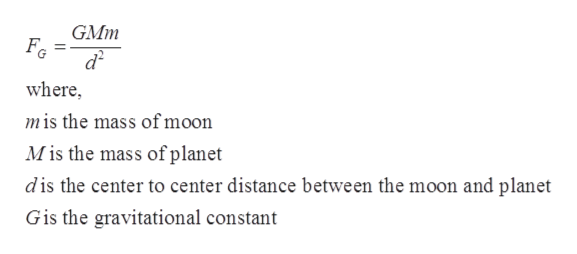# A moon of mass m and radius a is orbiting a planet of mass M and of radius b at a distance d (center-to-center) in a circular orbit. Derive an expression for the total mechanical energy E of the moon in terms of m, M, d and the gravitational constant G.

Question
10 views

A moon of mass m and radius a is orbiting a planet of mass M and of radius b at a distance d (center-to-center) in a circular orbit.

Derive an expression for the total mechanical energy E of the moon in terms of mMd and the gravitational constant G

check_circle

Step 1

Given:

Mass of moon = m

Mass of planet =  M

Distance between the center of moon and planet = d

Step 2

Since moon is rotating in the circular path. Therefore, centripetal force experienced by the moon ishelp_outlineImage Transcriptionclosemv? F. where, mis the mass of moon vis the velocity of moon dis the center to center distance between the moon and planet fullscreen
Step 3

Gravitational force experienced by...help_outlineImage TranscriptioncloseGMm Fe d? where, mis the mass of moon M is the mass of planet dis the center to center distance between the moon and planet Gis the gravitational constant fullscreen

### Want to see the full answer?

See Solution

#### Want to see this answer and more?

Solutions are written by subject experts who are available 24/7. Questions are typically answered within 1 hour.*

See Solution
*Response times may vary by subject and question.
Tagged in

### Gravitation